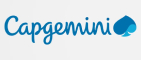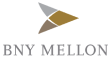New update is available. Click here to update.
Last Updated: 27 Jan, 2021

# Find Duplicate in Array

Easy+7 more companies

## Problem statement#### Note :

``````All the integers in the array appear only once except for precisely one integer which appears two or more times.
``````

#### Input format:

``````The first line of input contains an integer ‘T’ denoting the number of test cases. Then the T test cases follow.

The first line of each test case contains an integer ‘N’, the number of elements in the array.

The second line of each test case contains ‘N’ space-separated integers representing the elements of the array.
``````

#### Output format:

``````For each test case, the duplicate element of the given array is printed.

The output of each test case is printed in a separate line.
``````
##### Note :
``````You are not supposed to print anything; It has already been taken care of. Just implement the given function.
``````
##### Constraints :
``````1 <= T <= 5
1 <= N <= 10^5
1 <= ARR[i] <= N - 1

Time Limit: 1 sec
``````## Approaches

### 01 ApproachFirst, we sort the array. Now, the rest of the algorithm becomes fairly simple. We just compare each element to its previous element. As we know there is exactly one repeated element, hence we can simply just return the element, which is equal to its previous element as soon as we find it.